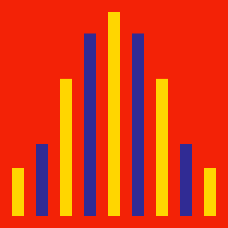Probability

# Discrete Probability Distribution - Uniform Distribution

Jill has a set of $33$ cards labelled with integers from 1 through $33.$ She faces all the cards down, shuffles the deck repeatedly and then picks the card on the top. What is the probability that the card she picks shows a number larger than $19?$

Note: No two cards have the same number.

Which of the following represents the probability density function of a random variable $X$ that takes on integers following the discrete uniform distribution $X\sim U\{9,48\}?$

Harry spins a roulette which consists of integers from 1 through $14$ (not necessarily in order). What is the variance of the outcome he gets?

Note: The areas that correspond to each number are equal.

Find the variance of a random variable $X$ which follows the discrete uniform distribution $X \sim U\{5,18\}?$

What is the expected value of the outcome when throwing a fair die?

×